Home » Everything you Should Know about Confusion Matrix for Machine Learning

# Everything you Should Know about Confusion Matrix for Machine Learning

## Confusion Matrix – Not So Confusing!

Have you been in a situation where you expected your machine learning model to perform really well but it sputtered out a poor accuracy? You’ve done all the hard work – so where did the classification model go wrong? How can you correct this?

There are plenty of ways to gauge the performance of your classification model but none have stood the test of time like the confusion matrix. It helps us evaluate how our model performed, where it went wrong and offers us guidance to correct our path.In this article, we will explore how a Confusion matrix gives a holistic view of the performance of your model. And unlike its name, you will realize that a Confusion matrix is a pretty simple yet powerful concept. So let’s unravel the mystery around the confusion matrix!Learning the ropes in the machine learning field? These courses will get you on your way:

## Here’s what we’ll cover:

• What is a Confusion Matrix?
• True Positive
• True Negative
• False Positive – Type 1 Error
• False Negative – Type 2 Error
• Why need a Confusion matrix?
• Precision vs Recall
• F1-score
• Confusion matrix in Scikit-learn
• Confusion matrix for multi-class Classification

## What is a Confusion Matrix?

The million dollar question – what, after all, is a confusion matrix?

A Confusion matrix is an N x N matrix used for evaluating the performance of a classification model, where N is the number of target classes. The matrix compares the actual target values with those predicted by the machine learning model. This gives us a holistic view of how well our classification model is performing and what kinds of errors it is making.

For a binary classification problem, we would have a 2 x 2 matrix as shown below with 4 values: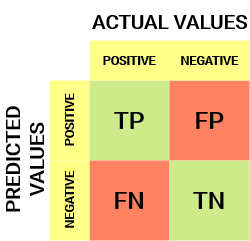Let’s decipher the matrix:

• The target variable has two values: Positive or Negative
• The columns represent the actual values of the target variable
• The rows represent the predicted values of the target variable

But wait – what’s TP, FP, FN and TN here? That’s the crucial part of a confusion matrix. Let’s understand each term below.

## Understanding True Positive, True Negative, False Positive and False Negative in a Confusion Matrix

True Positive (TP)

• The predicted value matches the actual value
• The actual value was positive and the model predicted a positive value

True Negative (TN)

• The predicted value matches the actual value
• The actual value was negative and the model predicted a negative value

False Positive (FP) – Type 1 error

• The predicted value was falsely predicted
• The actual value was negative but the model predicted a positive value
• Also known as the Type 1 error

False Negative (FN) – Type 2 error

• The predicted value was falsely predicted
• The actual value was positive but the model predicted a negative value
• Also known as the Type 2 error

Let me give you an example to better understand this. Suppose we had a classification dataset with 1000 data points. We fit a classifier on it and get the below confusion matrix: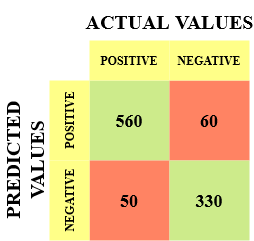The different values of the Confusion matrix would be as follows:

• True Positive (TP) = 560; meaning 560 positive class data points were correctly classified by the model
• True Negative (TN) = 330; meaning 330 negative class data points were correctly classified by the model
• False Positive (FP) = 60; meaning 60 negative class data points were incorrectly classified as belonging to the positive class by the model
• False Negative (FN) = 50; meaning 50 positive class data points were incorrectly classified as belonging to the negative class by the model

This turned out to be a pretty decent classifier for our dataset considering the relatively larger number of true positive and true negative values.

Remember the Type 1 and Type 2 errors. Interviewers love to ask the difference between these two!

## Why Do We Need a Confusion Matrix?

Before we answer this question, let’s think about a hypothetical classification problem.

Let’s say you want to predict how many people are infected with a contagious virus in times before they show the symptoms, and isolate them from the healthy population (ringing any bells, yet? 😷). The two values for our target variable would be: Sick and Not Sick.

Now, you must be wondering – why do we need a confusion matrix when we have our all-weather friend – Accuracy? Well, let’s see where accuracy falters.

Our dataset is an example of an imbalanced dataset. There are 947 data points for the negative class and 3 data points for the positive class. This is how we’ll calculate the accuracy: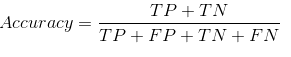Let’s see how our model performed: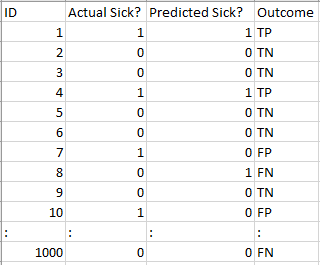The total outcome values are:

TP = 30, TN = 930, FP = 30, FN = 10

So, the accuracy for our model turns out to be: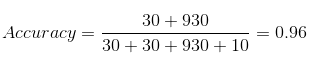But it is giving the wrong idea about the result. Think about it.

Our model is saying “I can predict sick people 96% of the time”. However, it is doing the opposite. It is predicting the people who will not get sick with 96% accuracy while the sick are spreading the virus!

Do you think this is a correct metric for our model given the seriousness of the issue? Shouldn’t we be measuring how many positive cases we can predict correctly to arrest the spread of the contagious virus? Or maybe, out of the correctly predicted cases, how many are positive cases to check the reliability of our model?

This is where we come across the dual concept of Precision and Recall.

## Precision vs. Recall

Precision tells us how many of the correctly predicted cases actually turned out to be positive.

Here’s how to calculate Precision: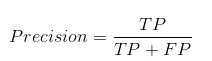This would determine whether our model is reliable or not.

Recall tells us how many of the actual positive cases we were able to predict correctly with our model.

And here’s how we can calculate Recall: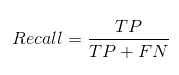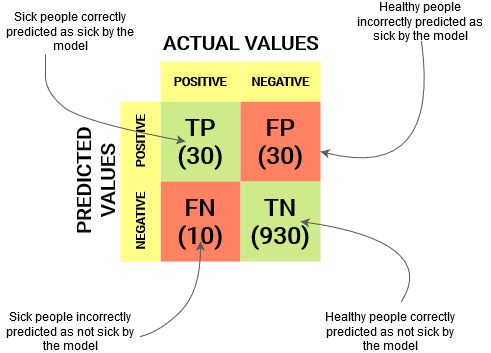We can easily calculate Precision and Recall for our model by plugging in the values into the above questions: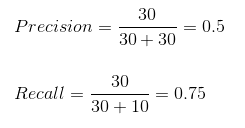50% percent of the correctly predicted cases turned out to be positive cases. Whereas 75% of the positives were successfully predicted by our model. Awesome!

Precision is a useful metric in cases where False Positive is a higher concern than False Negatives.

Precision is important in music or video recommendation systems, e-commerce websites, etc. Wrong results could lead to customer churn and be harmful to the business.

Recall is a useful metric in cases where False Negative trumps False Positive.

Recall is important in medical cases where it doesn’t matter whether we raise a false alarm but the actual positive cases should not go undetected!

In our example, Recall would be a better metric because we don’t want to accidentally discharge an infected person and let them mix with the healthy population thereby spreading the contagious virus. Now you can understand why accuracy was a bad metric for our model.

But there will be cases where there is no clear distinction between whether Precision is more important or Recall. What should we do in those cases? We combine them!

## F1-Score

In practice, when we try to increase the precision of our model, the recall goes down, and vice-versa. The F1-score captures both the trends in a single value: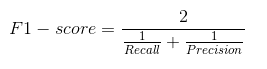F1-score is a harmonic mean of Precision and Recall, and so it gives a combined idea about these two metrics. It is maximum when Precision is equal to Recall.

But there is a catch here. The interpretability of the F1-score is poor. This means that we don’t know what our classifier is maximizing – precision or recall? So, we use it in combination with other evaluation metrics which gives us a complete picture of the result.

## Confusion Matrix using scikit-learn in Python

You know the theory – now let’s put it into practice. Let’s code a confusion matrix with the Scikit-learn (sklearn) library in Python.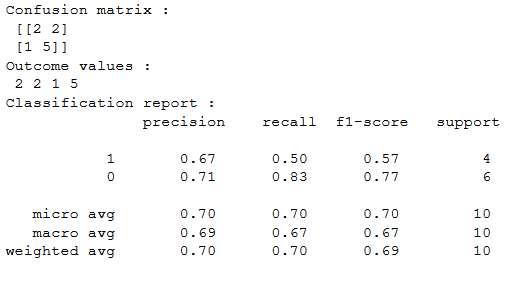Sklearn has two great functions: confusion_matrix() and classification_report().

• Sklearn confusion_matrix() returns the values of the Confusion matrix. The output is, however, slightly different from what we have studied so far. It takes the rows as Actual values and the columns as Predicted values. The rest of the concept remains the same.
• Sklearn classification_report() outputs precision, recall and f1-score for each target class. In addition to this, it also has some extra values: micro avg, macro avg, and weighted avg

Mirco average is the precision/recall/f1-score calculated for all the classes.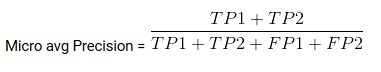Macro average is the average of precision/recall/f1-score.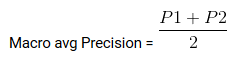Weighted average is just the weighted average of precision/recall/f1-score.

## Confusion Matrix for Multi-Class Classification

How would a confusion matrix work for a multi-class classification problem? Well, don’t scratch your head! We will have a look at that here.

Let’s draw a confusion matrix for a multiclass problem where we have to predict whether a person loves Facebook, Instagram or Snapchat. The confusion matrix would be a 3 x 3 matrix like this:The true positive, true negative, false positive and false negative for each class would be calculated by adding the cell values as follows: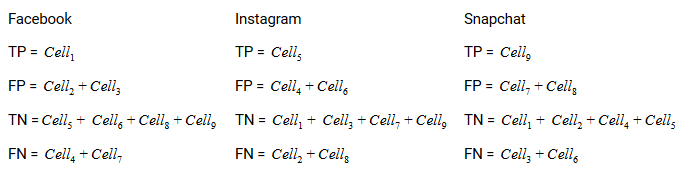That’s it! You are ready to decipher any N x N confusion matrix!

## End Notes

And suddenly the Confusion matrix is not so confusing any more! This article should give you a solid base on how to intepret and use a confusion matrix for classification algorithms in machine learning.

We will soon come out with an article on the AUC-ROC curve and continue our discussion there. Until next time, don’t lose hope in your classification model, you just might be using the wrong evaluation metric!

You can also read this article on our Mobile APP•Punit Kumar says:

Hi Aniruddha,

Thanks for writing this. Isn’t the definition of FP and FN other way around? Like
False Positive (FP) – The actual value was positive but we predicted a negative value
Shouldn’t it be reversed?

•Aniruddha Bhandari says:

Hey Punit,
Thanks for taking out the time to read the article and pointing out the mistake. Much appreciated!
Thanks
Aniruddha

•Prasad Karanjkar says:

Thanks Aniruddha-

•Ryan Tabeshi says:

Hi Puneet,

In a FP, the value was predicted to be positive, but the value actually belonged to the negative class, so I think its correct, unless I’m missing something.

•Ratheehsh says:

Thanks bro..You simply explained it..

•Arjun Badhan says:

Hi Aniruddha,

Thanks for the article. It is indeed informative.

However, I would like to highlight something in the section with heading “Understanding True Positive, True Negative, False Positive and False Negative in a Confusion Matrix”. Do you think that we might have mixed up on the second point on False Positive and False Negative.

•Aniruddha Bhandari says:

Hi Arjun,
Glad you found it useful. And you are correct in pointing out the mix-up in the definitions. Thanks for your timely intervention🙏.
Aniruddha

•Sagar says:

Nice and well written article Aniruddha. Introduced confusion matrix very well for beginners.

(But I think there is one minor issue you may want to correct if you also notice it is really an issue: Under Type1 and Type2 error definitions, I think you have to swap 2nd bullet points. These do not match with matrix you mentioned earlier.)

•Aniruddha Bhandari says:

Hey Sagar
Really glad you liked the article! I have made the relevant changes. Thanks for the feedback!
Aniruddha

•Luciano Caroti says:

I think that you mismatched the 2nd row in the definition of False positive with the 2nd row in the definition of false negative

•Aniruddha Bhandari says:

Hey Luciano
Aniruddha

•Shahnawaz Sayyed says:

Awesome!!! Very simple and crisp explaination. Thanks So much

•Aniruddha Bhandari says:

•Abid Saber says:

How can we extract Tp, FP, FN, TN from the confusion matrix in the multiclass case with sklearn

•Aniruddha Bhandari says:

Hi Abid
If you want to calculate the values for a multiclass classification problem, you will need to calculate it manually as Sklearn returns the value of the Confusion matrix and not really the TP, FP, etc. (which was in the case of binary classification as we only had two classes).
I have written the code for finding the TP, FP, etc. for one class of a multiclass classification problem here. The rest I am pretty sure you will be able to manage yourself. If not, feel free to ask your doubts.
Thanks
Aniruddha

•MRB says:

Aniruddha: Too often confusion matrices deserve their unfortunate name, especially for students just starting to learn ML etc. Thanks for sharing a helpful tutorial & correcting the typo. 😃. It always helps (me, anyhow) to see some ‘friendly’ examples which I can follow along by myself so I’m sure others are going to find your contribution worthwhile too.

•Trinkesh says:

great bro.